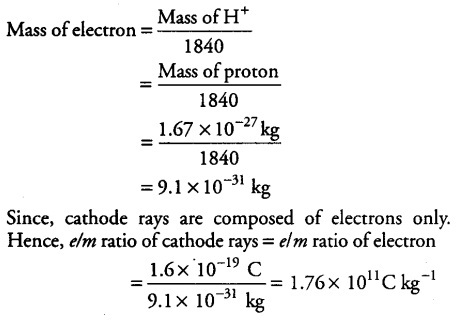# If the mass of a proton is 1.67x\${{10}^{-27}}\$ kg

If the mass of a proton is 1.67x\${{10}^{-27}}\$ kg and if electron is found to be 1840 times lighter than hydrogen ion. Then find the charge to mass ratio of cathode rays.
[Given, charge on 1 electron = 1.6 x\${{10}^{-19}}\$ C]